# Mechanical Wave Definition & Task Formulas

By: Max Malak

9 min

0

10.04.2022Many of you find physics homework to be boring. Yet this is not the case because everything happens precisely thanks to this science in real life. We can look at this natural science from the perspective of problem-solving and creating formulas. Today we will talk about the mechanical wave definition and tell you what formula to use to solve the task.

## Mechanical Wave - What Is It?

When throwing a stone into the water, look at the water waves. So you can watch the fantastic physical process and not waste time on empty fun.

Suppose in some medium (they are gaseous, solid, and liquid), the vibration activity of particles increases. This change occurs due to the interaction of atoms and molecules of the above environment. In that case, vibrations are produced that transfer data from one side to the other, adhering to a finite speed. This process is called a wave.

What happens to the float when it is on ocean waves? As the float rises and falls, the float follows the movement of the water as the waves pass it by. Thus, waves are called the process of propagation of oscillations in space from point to point, from particle to particle.

Mechanical waves are waves that are capable of propagating vibrations of various particles in space. The presence of elastic properties in a medium is necessary for wave propagation: deformation that occurs in any place due to the interaction of neighboring particles is successively transferred from one side of the medium to the other. Different types of deformations will correspond to different types of waves.

## Two Types Of Mechanical Waves

There are two types of mechanical waves: transverse waves and longitudinal waves. Consider them in more detail and see the amplitude, direction of the wave, motion of the wave, and pressure waves.

### Transverse Waves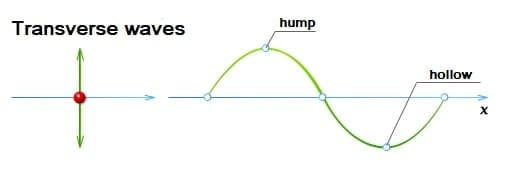A transverse wave is a wave that vibrates various particles in a medium in a perpendicular direction. In this case, the direction not depends on the propagation of the wave. Mechanical waves can be transverse only in a medium in which shear deformations are possible. Consequently, shear waves are not observed in liquids and gases. Transverse mechanical waves arise in solids. An example of such waves is the waves that propagate in the guitar string. The transverse wave has polarization. The amplitude vector of this wave has a certain orientation in the transverse plane.

#### Shear Speed Of The Transverse Wave

You can find the speed of propagation of transverse waves in an infinite isotropic medium using the formula:

In this formula: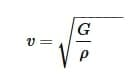• G is the shear modulus of the medium;
• p waves are the density of the substance.

The elastic properties and density of a solid depend on the substance's chemical composition, and it changes insignificantly with changes in pressure and temperature. Therefore, in most cases, the speed of wave propagation can be considered constant. The speed in the formula is called the phase speed.

### Longitudinal Waves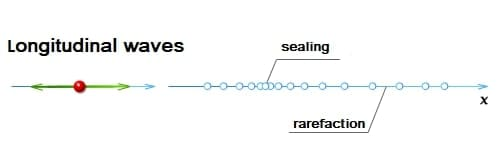A longitudinal wave is a wave that moves and spreads particles depending on the direction of the wave. Longitudinal waves propagate in a substance, in which elastic forces arise, during tensile and compression deformation in a substance in any aggregation state.

So, consider an example of a longitudinal wave. Sound waves are waves that sound in the air and are classified as longitudinal waves. Longitudinal waves that propagate frequencies from 17 to 20 ~ 000 Hz are known as sound waves. The speed of propagation of acoustic waves depends on the properties of the medium and its temperature.

When a given wave propagates in a particular medium, alternations of thickening and rarefaction of particles occur, moving in the direction of wave propagation with a velocity v. All the time the wave exists, the elements of the medium perform oscillations at their equilibrium positions. In contrast, different particles oscillate with a phase shift. In solids, the speed of wave travels is greater than the speed of shear waves.

#### Longitudinal Wave Propagation Speed

The propagation speed of longitudinal elastic waves in homogeneous gases or liquids is equal to:

In this formula: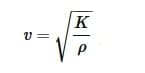• K is the bulk modulus of the substance;
• ρ = const is the density of the medium. In gases, the formula is valid if the excess pressure is much less than the equilibrium pressure waves of the undisturbed gas.

The propagation speed of longitudinal waves in a thin rod caused by its longitudinal tension and compression is equal to: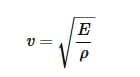In this formula:

• E - Young's modulus of the rod substance.
• ρ = const is the density of the medium.

## Longitudinal and Transverse Wave Equation

The main task in studying waves is to establish the law of change in time and space of physical quantities. When considering elastic waves, such a quantity is, for example, the displacement (s waves) of different particles, depending on placement and balance. The function s depending on the coordinates of space and time is called the wave equation.

Harmonic waves are the simplest and most well-known type of waves. In such waves, the parameters s for all particles of the medium create harmonic oscillations. In this case, the frequencies will be the same. To implement this wave process, the source of harmonic waves must perform continuous harmonic oscillations.

Let a one-dimensional shear wave propagate along the X-axis from a wave source located at the origin of coordinates - point O. The most famous example of such a wave is the infinite elastic string, which has an oscillating end. If oscillations at point O occur according to the law: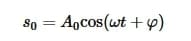In this formula:

• A0 - amplitude;
• ω - cyclic vibration frequency;
• φ is the initial phase.

Then the oscillations at some arbitrary point A on the X-axis lag behind s0 in-phase and occur according to the law: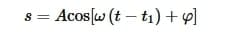In this formula:

• t1 = x / v - the time it takes for the wave passes the distance from the wave source to the considered point A (OA = x).
• A is the amplitude of the wave at point A.

If a specific medium, which is located in the wave, does not absorb energy, then the amplitudes of the oscillations and the wave's amplitude coincide.

## Task Examples Of Mechanical Waves

Tasks for calculating wave moves, the direction of motion waves, transfers energy, transport energy are found not only in physics but also in math homework. Therefore, we will consider examples of how to cope with these types of tasks.

### Example 1

Task: There is a one-dimensional wave, while it is transverse and has a period T and an oscillation amplitude A. It propagates with speed v. What is the displacement of a particle of the medium, which is at a distance x1 from the wave source at time t1 from the beginning of oscillations? Draw the considered wave propagating along the X-axis for a fixed point in time (t1).

Decision: Let's formulate a one-dimensional wave, which will give us the displacement of a particle of the medium: s = Acos [ωt - kx + φ].

We will assume that the initial phase of oscillations is equal to zero (φ = 0). We find the cyclic frequency, knowing the period of oscillation of points in the wave: ω = 2π / T.

The wavenumber is: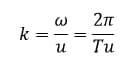Let's rewrite the wave equation: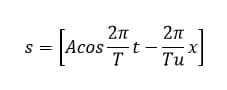To find the displacement of the point specified in the task statement, we substitute these parameters into the equation: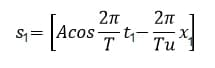From the received data, we can draw a wave: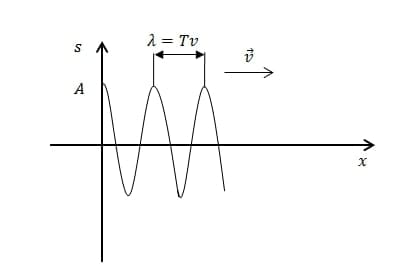### Example 2

Task: A one-dimensional plane wave propagates in an elastic medium. Draw on the graph the direction of the velocity of the medium particles at the points s = 0, at t = 0 for longitudinal and transverse waves.

Decision: The equation of a one-dimensional plane wave is the expression: s = Acos [ωt − kx]. At t = 0 from the expression we get: s = Acos [kx].

In a longitudinal wave, particles are displaced along the direction of the wave velocity: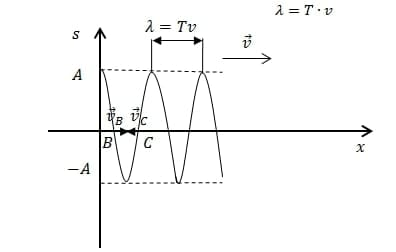In a longitudinal wave, particles oscillate across the direction of the wave velocity: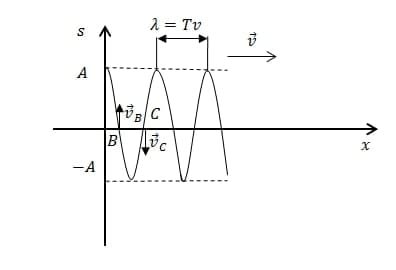## What Else Do You Need To Know About Mechanical Waves?

According to science homework, you may come across different terms, so here is a list of definitions that come in handy in completing an assignment:

• surface waves are waves that occupy a position along with the interface between two media and penetrate these media at a distance less than the wavelength;
• seismic waves, scientists call waves propagating in the Earth from earthquake foci or any powerful explosions;
• electromagnetic waves are electromagnetic oscillations that propagate in space at a finite speed.

User ratings:

User ratings is 4.1 stars.Max Malak

Product Manager

Here at Studybay, I work as a Head of Affiliates in the marketing department. I studied Liberal Arts and took related classes at Tokyo Sophia University. I believe that challenges are what make my job fun and exciting. That's why I like completing complex, complicated, and even weird tasks and then sharing my experience with colleagues.